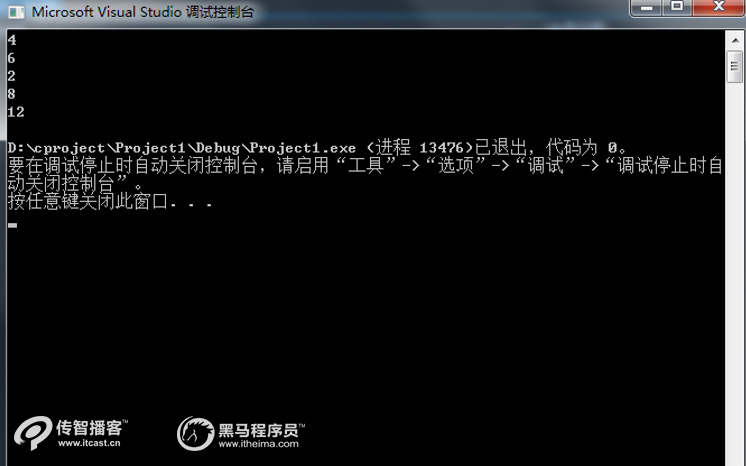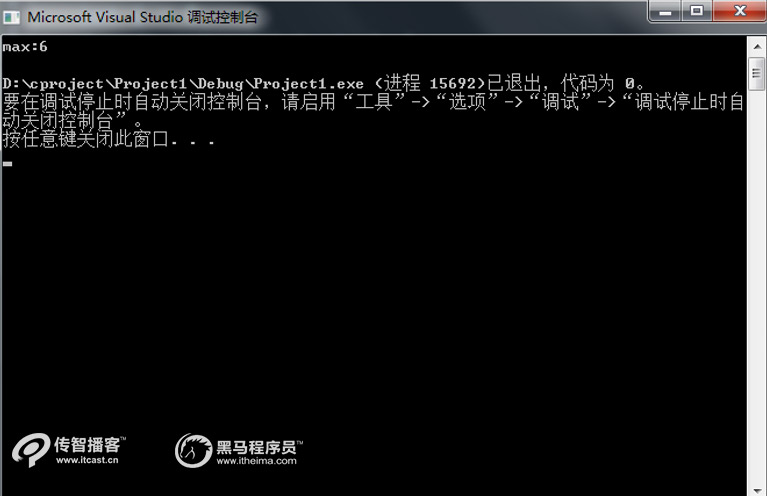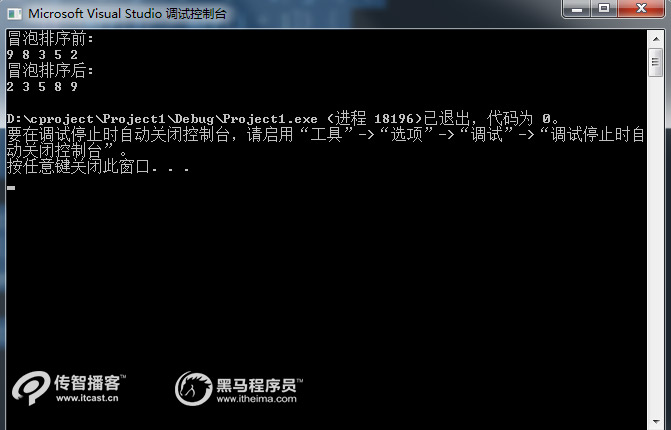# www.8029.com-首页### 全国校区## C++中一维数组是什么？怎么使用？【C++培训】

int array[5l;

（1）直接对数组中的所有元素赋值，示例代码如下：

int i={1,2,3,4,5};

（2）只对数组中的一部分元素赋值，示例代码如下

int i={1,2,3};

（3）对数组全部元素赋值，但不指定长度，示例代码如下：

int i[]={1,2,3,4};

（1）数组的下标是用方括号括起来的，而不是圆括号；

（2）数组名的命名同变量名的命名规则相同；

（3）数组定义中，常量表达式的值可以是符号常量，如下面的定义就是合法的。

int a[N];      //假设预编译命令#define N 4，下标是符号常量

#include

void main(int argc, char *argv[]){

int x = {2,3,1,4,6};

int i;

for (i = 0; i < 5; i++) {

printf("%d\n", 2 * x[i]);

}

}1、一维数组的遍历

#include

void main(int argc, char *argv[]){

int x = {2,3,1,4,6};

int i = 0;

for (i = 0; i < 5; i++) {

printf("x[%d]:%d\n", i, x[i]);

}

}2、一维数组的最值

#include

int main(int argc, char *argv[]){

int x = {2,3,1,4,6};

int nMax = x;

int i = 0;

for (i = 0; i < 5; i++) {

if (x[i] > nMax) {

nMax = x[i];

}

}

printf("max:%d\n", nMax);

return 0;

}3、一维数组的排序

#include

void main(int argc, char ** argv[]){

int arr = { 9,8,3,5,2 };

int temp = 0;

int i, j;

printf("冒泡排序前:\n");

for (i = 0; i < 5; i++) {

printf("%d ", arr[i]);

}

printf("\n");

for (i = 0; i < 5 - 1; i++) {

for (j = 0; j < 5 - 1 - i; j++) {

if (arr[j] > arr[j + 1]) {

temp = arr[j];

arr[j] = arr[j + 1];

arr[j + 1] = temp;

}

}

}

printf("冒泡排序后:\n");

for (i = 0; i < 5; i++) {

printf("%d ", arr[i]);

}

printf("\n");

}C语言如何获取随机数?
C/C :指针数组和数组指针浅析
C语言二维数组的定义和引用

## 相关阅读javaeepythonwebuicloudtestnetmarketPython